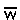Derivation of the General Selection Equation
______________________________________________________________

Genotype         AA                  AB                BB
______________________________________________________________

(1) Frequency     p2            +    2pq            +    q2             =               1
before selection

(2)  Fitness         W0                  W1                 W2

(3) Relative        p2W0        +    2pqW1       +    q2W2        =Contribution

(4) Frequency    p2W0/+   2pqW1/+    q2W2/=/= 1
after selection
______________________________________________________________

(1) Genotype distributions before selection follow Hardy-Weinberg expectations.

(2) Each genotype AA, AB, and BB has a distinct phenotype: W0, W1, & W2, respectively.
W is the expectation that an individual with a particular genotype will survive & reproduce

(3) Each genotypic class makes a relative contribution to the next generation,
which is proportional to its initial frequency, weighted by its fitness.

[e.g., if the AA genotype has a frequency of 0.25 and 80% survive to reproduce,
the relative contribution of AA to the next generation is (0.25)(0.8)=0.20]

The sum of the relative contributions of all three genotypes is(read as, "W bar") = mean population fitness
In this simple model,< 1, because not everyone on Line (1) survives.

(4) Because< 1, the relative contributions have to be "normalized":
Dividing the contribution of each genotype byreturns the sum to unity,
and the final values are the relative genotype frequencies after selection.

To derive the allele frequencies after selection, take Line (4) above and

recall  q = f(BB) + (1/2) f(AB)

so  q' =  q2W2/+ (1/2) 2pqW1/=  q(qW2 + pW1)/thenq   =  q' - q  = qafter - qbefore

=  q(qW2 + pW1)/-  q/=  [(q)(qW2 + pW1) - (q)(p2W0 + 2pqW1 + q2W2)] /=  [(q)(qW2 + pW1 - p2W0 - 2pqW1 - q2W2)] /=  [(q)(pqW2 + W1p(1-2q) - pW0p)] /[Note 1]

=  [(pq)(qW2 + W1(1-2q) - W0p)] /=  [(pq)(W2q + W1(p-q) - W0p)] /[Note 2]

=  [(pq)(W2q + W1p - W1q - W0p)/q  =  [pq] [(q)(W2 - W1) + (p)(W1 - W0)] / []
________________________________________________

Notes:   (q - q2) = q(1 - q) = pq          (1 - 2q) = (1 - q) - q = p - q

Homework
:  repeat this derivation forp = p' - p

Text material © 2022 by Steven M. Carr